# DAV Class 5 Maths Chapter 14 Brain Teasers Solutions

The DAV Books Solutions Class 5 Maths and DAV Class 5 Maths Chapter 14 Brain Teasers Solutions of Bills offer comprehensive answers to textbook questions.

## DAV Class 5 Maths Ch 14 Brain Teasers Solutions

Question 1.
(a) What is a bill?
Solution:
Bill is a slip of paper on which a shopkeeper notes down the requirements of a customer and calculates the total cost of items purchased.

(b) How is the bill useful in case of a defective item?
Solution:
A customer can exchange it easily by showing the bill.

Question 2.
You are given a bill below. Fill in the blanks: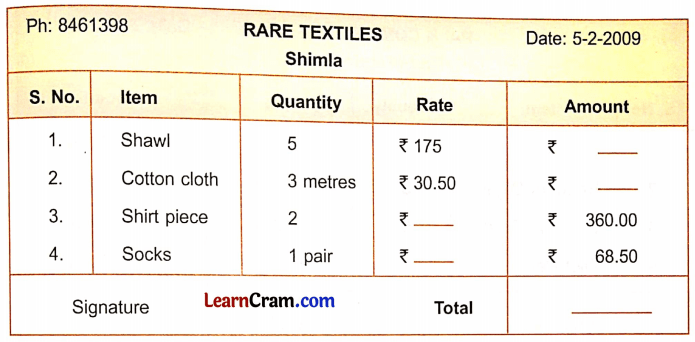Solution:
1. 5 × ₹ 175 = ₹ 875

2. 3 × ₹ 30.50 = ₹ 91.50

3. 360.00 ÷ 2 = 180.00

4. ₹ 68.50

Total = (₹ 875 + ₹ 91.50 + ₹ 360 + ₹ 68.50) = ₹ 1,395Question 3.
Rahul got the following bill from the Raj Sweet Shop. Rahul finds some errors in this bill. Find out those errors.Solution:
The amount calculation of Pista Burfi is wrong. It should be ₹ 360.
The amount calculation of Jalebi is wrong. It should be ₹ 240.
The amount calculation of Gulab Jamun is wrong. It should be ₹ 560.
Hence calculation of the total is wrong.
Right total amount = ₹ 2,150

Question 4.
Look carefully at the bill given below and answer the questions that follow: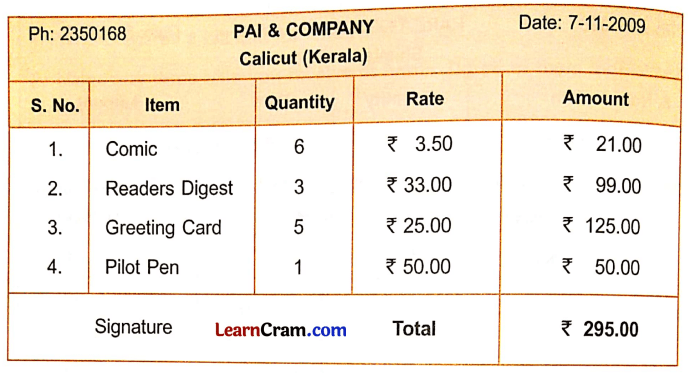(a) From which shop were the above items purchased and when were they purchased?
Solution:
Pai & Company, On 7 November 2014

(b) Where is this shop located?
Solution:
Calicut (Kerala)

(c) How many items were purchased from Pai & Company?
Solution:
4 items

(d) What is the cost of one comic?
Solution:
₹ 30

(e) What is the cost of five greeting cards?
Solution:
₹ 125

(f) If I want to buy three pilot pens, how much should I pay?
Solution:
₹ 150

(g) What is the total cost of the items purchased?
Solution:
₹ 360Question 5.
Anil purchased the following items from the Aggarwal Namkeen Comer on 6 July 2014. Prepare a bill for these items. If Anil gave the shopkeeper one hundred rupee note, how much balance would he get back?
(a) Three packets of potato chips at ₹ 20.50 per packet.
(b) Two packets of cream biscuits at ₹ 10.50 per packet.
(c) Six pastries at ₹ 35 per pastry.
(d) One Pepsi for ₹ 20.
Solution:If he gave a 100 rupee note, then he has to give ₹ 212.50 more to clear the balance.

Question 6.
Prepare a bill for the following items purchased from Raj General Store, Paschim Vihar, Delhi on 11 January 2014. Find the total amount to be paid.
(a) 1$$\frac{1}{2}$$ kg sugar for ₹ 60.
(b) 500 grams urad dal for ₹ 45.50.
(c) One bottle of jam for ₹ 95.00.
(d) Five packets of biscuits for ₹ 125.
Solution: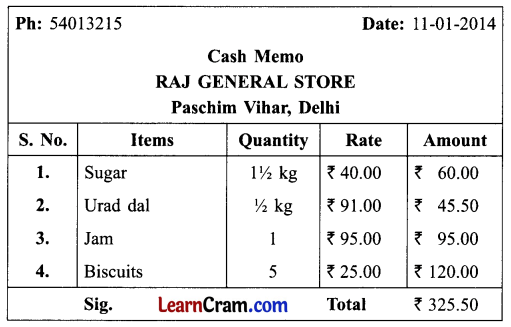Total amount to be paid = ₹ 325.50.

Question 1.
Name different types of bills.
Solution:
Gas bill, electricity bill, water bill, ration bill, mobile bill, etc.

Question 2.
Write the steps to prepare a bill.
Solution:

• Step 1: Write the name of the shop, its location, phone number, and date of purchase of items.
• Step 2: Make five columns – item number, name of item, quantity, rate, and amount.
• Step 3: Enter the name of the item, rate, and quantity.
• Step 4: We calculate the amount for each item.
• Step 5: We find the total cost of items purchased.Question 3.
Look carefully at the bill given below and answer the questions that follow: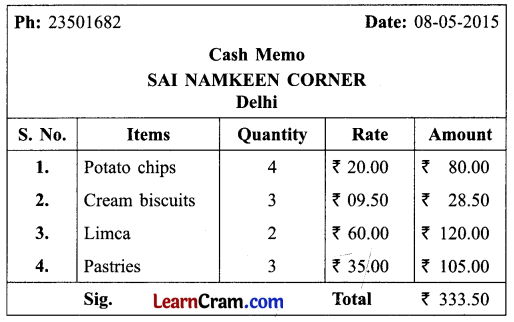(a) From which shop were the above items purchased and when they were purchased?
Solution:
Sai Namkeen Corner, On 8th May 2015.

(b) Where is the shop located?
Solution:
It is located in Delhi.

(c) How many items were purchased?
Solution:
Four items were purchased.

(d) What is the cost of 1 pastry?
Solution:
Cost of 1 pastry = ₹ 35.

(e) What is the total cost of items purchased?
Solution:
Total cost = ₹ 333.50

(f) If I want to buy 4 pastries, how much should I pay?
Solution:
Cost of 4 pastries = 4 × ₹ 35 = ₹ 140

Question 4.
Fill in the blanks and complete the bill.Solution:
Amount of book = 1 × ₹ 170 = ₹ 170
Amount of Note books = 10 × ₹ 7.50 = ₹ 75
Total Amount = ₹ 162 + ₹ 220 + ₹ 170 + ₹ 75 = ₹ 627

Question 5.
Find out the errors in the following bill.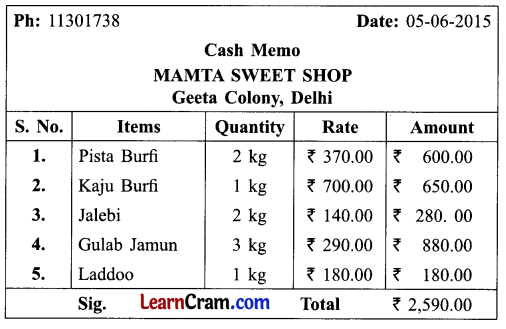Solution:
The amount of Pista Burfi is wrong, the amount of Kaju Burfi is wrong, amount of Gulab Jamun is wrong so, the total is also wrong.Question 6.
Prepare a bill for the following items purchased from Manik General Store, Dilshad Garden, Delhi on 25 June 2015. Find the total amount to be paid.
(a) 1$$\frac{1}{2}$$ kg Arhar dal for ₹ 120.
(b) 500 gm chana dal for ₹ 45.50.
(c) One bottle of ketchup for ₹ 110.00.
(d) Two packets of soap for ₹ 40.
Solution:Total amount to be paid = ₹ 315.50